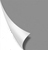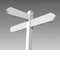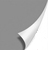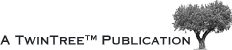#### 02-03 The Boltzmann Distributionhen a system has two discrete energy levels, there will be a well-defined pro­ba­bi­li­ty for finding a particle in the high unstable or the low, more stable energy level.

If we call the number of nuclei in the upper level Nu and that of nuclei in the lower level Nl, then the distribution between these two levels is given by the Boltzmann Dis­tri­bu­tion:

Nl / Nu = exp (ΔE / kT)

where ΔE is the energy difference between the two levels (ΔE = h × ω; h = 6.62 × 10-34 J × s); k is the Boltzmann constant (k = 1.3181 × 10-23J × K-1); and T is the absolute tem­pe­ra­ture (Kelvin).

It is clear from this formula that, at equilibrium, the larger the energy dif­fe­ren­ce ΔE becomes, the larger will be the population difference.

The energy difference between the two energy levels in the magnetic re­so­nan­ce ex­pe­ri­ment is proportional to the field strength. Thus, if we increase the field strength we increase the energy difference and hence also the population dif­fe­ren­ce. Since the size of the NMR signal is directly dependent on the population difference, the signal also increases.

This explains the increase of the signal-to-noise ratio with field strength (Chapter 9 and Chapter 10).

Radiowaves can be considered as packets of energy. If the energy of such a packet equals ΔE, it will cause a spin to jump to the high energy level. After the ra­dio­fre­quen­cy (RF) pulse, we no longer have an equilibrium. To return to this state, the same num­ber of spins which jumped to the higher energy state have to return to the low level. In doing so, they will emit an amount of energy ΔE which corresponds to the signal in an NMR experiment.

The transitions back to the equilibrium do not occur immediately, but over a period of time following the RF pulse.